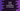# Swift String interpolation in 5 minutes## Swift String interpolation in 5 minutes :

Interpolation is the process to insert variables, constants, expressions, and literals into a string. Creating a custom string becomes easy with string interpolation. It works with both single line and multiline strings.

### Plus(+) to join strings and why we don’t use it :

You can use + to join multiple strings. For example :

``````let str1 = "Hello"
let str2 = "World"
let str3 = "!!"

let finalString = str1 + str2 + str3

print(finalString)``````

It will join all str1, str2, and str3 and creates the finalString as HelloWorld!!

But, for that, it will join str1 with str2 and create one string, join that string with str3 to produce the final string. That is a long operation and it creates lots of garbage variables. Also, you can’t join any string with other integer or long or any other data type.

String interpolation makes it easier. Even, we can add logical conditions while building the string.

## Example of string literal :

You can insert different types of items to a string literal. For that, you need to wrap that item in a pair of parentheses, prefixed by a backslash().

For example :

``````let boysMsg = "Hello Boys.."
let girlsMsg = "Hello Girls.."

let boy = true
let number = 30

let finalMsg = "\(boy ? boysMsg : girlsMsg). If you multiply \(number) with \(number), you will get \(Double(number * number))"

print(finalMsg)``````

We are creating the string finalMsg using string literal.

• We have one conditional statement that checks the current value of boy and adds different string based on it
• number is used in two places. We are also inserting the result of numbernumber* .

It prints the below string :

``Hello Boys... If you multiply 30 with 30, you will get 900.0``

## Extended string delimiter :

() is considered for string literal. If you don’t want to treat it as string literal, you can use extended string delimiter :

``````let msg = #"I am printing this \(message)"#
print(msg)``````

It will print :

``I am printing this \(message)``

We can also use string literal with it :

``````let msg = #"I am printing this \(message) and \#(2*2)"#
print(msg)``````

It will print :

``I am printing this \(message) and 4``